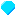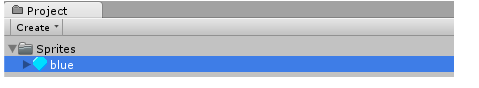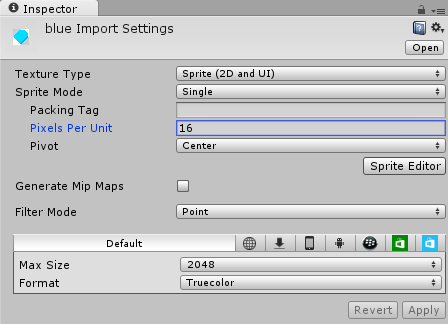# 【Unity3D开发小游戏】宝石迷阵

@[TOC]

## 二、资源下载

UI资源和源代码请搜索QQ群：1040082875下载

## 三、正文

### 2、宝石导入到我们项目中，Sprites文件夹：然后修改设置：注：Pixels Per Unit：16这意味着16x16像素将适合在游戏世界的一个单位。我们将把这个价值用于我们所有的宝石。其他的值如Filter Mode主要是为了平衡压缩和质量。

``````using UnityEngine;
using System.Collections;

public class Gem : MonoBehaviour {

// Use this for initialization
void Start () {

}

// Update is called once per frame
void Update () {

}
}
``````

``````using UnityEngine;
using System.Collections;

public class Gem : MonoBehaviour {

// Update is called once per frame
void Update () {

}
}
``````

``````public bool sameType(Gem other) {
return GetComponent<SpriteRenderer>().sprite == other.GetComponent<SpriteRenderer>().sprite;
}
``````

### 3、网格

``````using UnityEngine;
using System.Collections;

public class Grid : MonoBehaviour {

// Use this for initialization
void Start () {

}

// Update is called once per frame
void Update () {

}
}
``````

``````using UnityEngine;
using System.Collections;

public class Grid : MonoBehaviour {

// Update is called once per frame
void Update () {

}
}
``````

``````using UnityEngine;
using System.Collections;

public class Grid : MonoBehaviour {
// Grid size
public static int w = 8;
public static int h = 8;

// Update is called once per frame
void Update () {

}
}
``````

### 4、gemAt函数

``````public static Gem gemAt(float x, float y) {
// Find Gem at Position (x, y)
Gem[] gems = GameObject.FindObjectsOfType<Gem>();
foreach (Gem g in gems)
if ((Vector2)g.transform.position == new Vector2(x, y))
return g;
return null;
}
``````

### 5、生成宝石

``````public class Grid : MonoBehaviour {
// Grid size
public static int w = 8;
public static int h = 8;

// Available Gems
public GameObject[] gems;
...
``````

``````void spawnAt(float x, float y) {
int index = Random.Range(0, gems.Length);
Instantiate(gems[index], new Vector2(x, y), Quaternion.identity);
}
``````

``````// Update is called once per frame
void Update () {
// Spawn Gem in empty spaces of top row
for (int x = 0; x < w; ++x)
if (!gemAt(x, h-1))
spawnAt(x, h-1);
}
``````

### 6、使宝石下落

``````// Update is called once per frame
void Update() {
// Fall downwards
float x = transform.position.x;
float y = transform.position.y;

while (y > 0 && !Grid.gemAt(x, y-1))
--y;
transform.position = new Vector2(x, y);
}
``````

### 7、寻找匹配

``````public static List<Gem> matchesAt(float x, float y) {
List<Gem> res = new List<Gem>();

// ToDo: check horizontal matches
// ToDo: check vertical matches

return res;
}
``````

``````// check horizontally
if same type at (x+1,y):
if same type at (x+2, y):
if same type at (x-1,y):
if same type at (x-2, y):
if >= 3 matches: add them to result;
``````

``````// check vertically
if same type at (x,y+1):
if same type at (x, y+2):
if same type at (x,y-1):
if same type at (x, y-2):
if >= 3 matches: add them to result;
``````

``````public static List<Gem> matchesAt(float x, float y) {
List<Gem> res = new List<Gem>();
Gem center = Grid.gemAt(x, y);

// check horizontally
List<Gem> hor = new List<Gem>();
if (Grid.gemAt(x+1, y) && Grid.gemAt(x+1, y).sameType(center)) {
if (Grid.gemAt(x+2, y) && Grid.gemAt(x+2, y).sameType(center))
}
if (Grid.gemAt(x-1, y) && Grid.gemAt(x-1, y).sameType(center)) {
if (Grid.gemAt(x-2, y) && Grid.gemAt(x-2, y).sameType(center))
}

// check vertically
List<Gem> ver = new List<Gem>();
if (Grid.gemAt(x, y+1) && Grid.gemAt(x, y+1).sameType(center)) {
if (Grid.gemAt(x, y+2) && Grid.gemAt(x, y+2).sameType(center))
}
if (Grid.gemAt(x, y-1) && Grid.gemAt(x, y-1).sameType(center)) {
if (Grid.gemAt(x, y-2) && Grid.gemAt(x, y-2).sameType(center))
}

return res;
}
``````

### 8、解决匹配

``````public static void solveMatches(List<Gem> matches) {
foreach (Gem g in matches)
Destroy(g.gameObject);
}
``````

``````solveMatches(matchesAt(x, y));
``````

``````// Update is called once per frame
void Update() {
float x = transform.position.x;
float y = transform.position.y;

// Any falling to do?
if (y > 0 && !Grid.gemAt(x, y-1)) {
// Fall downwards
while (y > 0 && !Grid.gemAt(x, y-1))
--y;
transform.position = new Vector2(x, y);

// Solve possible matches
Grid.solveMatches(Grid.matchesAt(x, y));
}
}
``````

``````// Update is called once per frame
void Update () {
// Spawn Gem in empty spaces of top row
for (int x = 0; x < w; ++x) {
if (!gemAt(x, h-1)) {
// Spawn
spawnAt(x, h-1);

// Something below? Then solve matches
if (gemAt(x, h-2))
solveMatches(matchesAt(x, h-1));
}
}
}
``````

### 9、宝石选择

``````using UnityEngine;
using System.Collections;

public class Select : MonoBehaviour {

// Use this for initialization
void Start () {

}

// Update is called once per frame
void Update () {

}
}
``````

``````using UnityEngine;
using System.Collections;

public class Select : MonoBehaviour {

// Update is called once per frame
void Update () {

}
}
``````

``````sing UnityEngine;
using System.Collections;

public class Select : MonoBehaviour {
// Current selection
float x = 0;
float y = 0;

// Visual Indicator
public Transform selectRect;

// Update is called once per frame
void Update () {

}
}
``````

``````using UnityEngine;
using System.Collections;

public class Select : MonoBehaviour {
// Current selection
float x = 0;
float y = 0;

// Visual Indicator
public Transform selectRect;

// Update is called once per frame
void Update () {
// Set the Indicator position
selectRect.position = new Vector2(x, y);
}
}
``````

``````// Update is called once per frame
void Update () {
// Get Gem at (x, y)
Gem selected = Grid.gemAt(x, y);
if (selected != null) {
if (Input.GetKeyDown(KeyCode.UpArrow) && Grid.gemAt(x, y+1))
++y;
else if (Input.GetKeyDown(KeyCode.DownArrow) && Grid.gemAt(x, y-1))
--y;
else if (Input.GetKeyDown(KeyCode.RightArrow) && Grid.gemAt(x+1, y))
++x;
else if (Input.GetKeyDown(KeyCode.LeftArrow) && Grid.gemAt(x-1, y))
--x;
}

// Set the Indicator position
selectRect.position = new Vector2(x, y);
}
``````

### 10、交换宝石

``````// Update is called once per frame
void Update () {
// Get Gem at (x, y)
Gem selected = Grid.gemAt(x, y);
if (selected != null) {
if (Input.GetKeyDown(KeyCode.UpArrow) && Grid.gemAt(x, y+1)) {
if (Input.GetKey(KeyCode.RightShift)) {
// ToDo check if valid swap
// ToDo swap and solve if it was valid
} else ++y;
} else if (Input.GetKeyDown(KeyCode.DownArrow) && Grid.gemAt(x, y-1)) {
if (Input.GetKey(KeyCode.RightShift)) {
// ToDo check if valid swap
// ToDo swap and solve if it was valid
} else --y;
} else if (Input.GetKeyDown(KeyCode.RightArrow) && Grid.gemAt(x+1, y)) {
if (Input.GetKey(KeyCode.RightShift)) {
// ToDo check if valid swap
// ToDo swap and solve if it was valid
} else ++x;
} else if (Input.GetKeyDown(KeyCode.LeftArrow) && Grid.gemAt(x-1, y)) {
if (Input.GetKey(KeyCode.RightShift)) {
// ToDo check if valid swap
// ToDo swap and solve if it was valid
} else --x;
}
}

// Set the Indicator position
selectRect.position = new Vector2(x, y);
}
``````

``````void swap(Gem a, Gem b) {
// Swap their positions
Vector2 temp = a.transform.position;
a.transform.position = b.transform.position;
b.transform.position = temp;
}

bool validSwap(Gem a, Gem b) {
// A swap is valid if it results in matches
swap(a, b);
bool res = Grid.matchesAt(a.transform.position.x, a.transform.position.y).Count > 0 ||
Grid.matchesAt(b.transform.position.x, b.transform.position.y).Count > 0;
swap(a, b);
return res;
}
``````

``````// Update is called once per frame
void Update () {
// Get Gem at (x, y)
Gem selected = Grid.gemAt(x, y);
if (selected != null) {
if (Input.GetKeyDown(KeyCode.UpArrow) && Grid.gemAt(x, y+1)) {
if (Input.GetKey(KeyCode.RightShift)) {
if (validSwap(selected, Grid.gemAt(x, y+1))) {
// ToDo swap and solve if it was valid
}
} else ++y;
} else if (Input.GetKeyDown(KeyCode.DownArrow) && Grid.gemAt(x, y-1)) {
if (Input.GetKey(KeyCode.RightShift)) {
if (validSwap(selected, Grid.gemAt(x, y-1))) {
// ToDo swap and solve if it was valid
}
} else --y;
} else if (Input.GetKeyDown(KeyCode.RightArrow) && Grid.gemAt(x+1, y)) {
if (Input.GetKey(KeyCode.RightShift)) {
if (validSwap(selected, Grid.gemAt(x+1, y))) {
// ToDo swap and solve if it was valid
}
} else ++x;
} else if (Input.GetKeyDown(KeyCode.LeftArrow) && Grid.gemAt(x-1, y)) {
if (Input.GetKey(KeyCode.RightShift)) {
if (validSwap(selected, Grid.gemAt(x-1, y))) {
// ToDo swap and solve if it was valid
}
} else --x;
}
}

// Set the Indicator position
selectRect.position = new Vector2(x, y);
}
``````

``````void swapAndSolve(Gem a, Gem b) {
swap(a, b);
Grid.solveMatches(Grid.matchesAt(a.transform.position.x, a.transform.position.y));
Grid.solveMatches(Grid.matchesAt(b.transform.position.x, b.transform.position.y));
}
``````

``````// Update is called once per frame
void Update () {
// Get Gem at (x, y)
Gem selected = Grid.gemAt(x, y);
if (selected != null) {
if (Input.GetKeyDown(KeyCode.UpArrow) && Grid.gemAt(x, y+1)) {
if (Input.GetKey(KeyCode.RightShift)) {
if (validSwap(selected, Grid.gemAt(x, y+1))) {
swapAndSolve(selected, Grid.gemAt(x, y+1));
++y;
}
} else ++y;
} else if (Input.GetKeyDown(KeyCode.DownArrow) && Grid.gemAt(x, y-1)) {
if (Input.GetKey(KeyCode.RightShift)) {
if (validSwap(selected, Grid.gemAt(x, y-1))) {
swapAndSolve(selected, Grid.gemAt(x, y-1));
--y;
}
} else --y;
} else if (Input.GetKeyDown(KeyCode.RightArrow) && Grid.gemAt(x+1, y)) {
if (Input.GetKey(KeyCode.RightShift)) {
if (validSwap(selected, Grid.gemAt(x+1, y))) {
swapAndSolve(selected, Grid.gemAt(x+1, y));
++x;
}
} else ++x;
} else if (Input.GetKeyDown(KeyCode.LeftArrow) && Grid.gemAt(x-1, y)) {
if (Input.GetKey(KeyCode.RightShift)) {
if (validSwap(selected, Grid.gemAt(x-1, y))) {
swapAndSolve(selected, Grid.gemAt(x-1, y));
--x;
}
} else --x;
}
}

// Set the Indicator position
selectRect.position = new Vector2(x, y);
}
``````

• 独特的图形风格
• 好看的背景
• 得分
• 保存和加载游戏
• 特殊事件
• 多重关卡